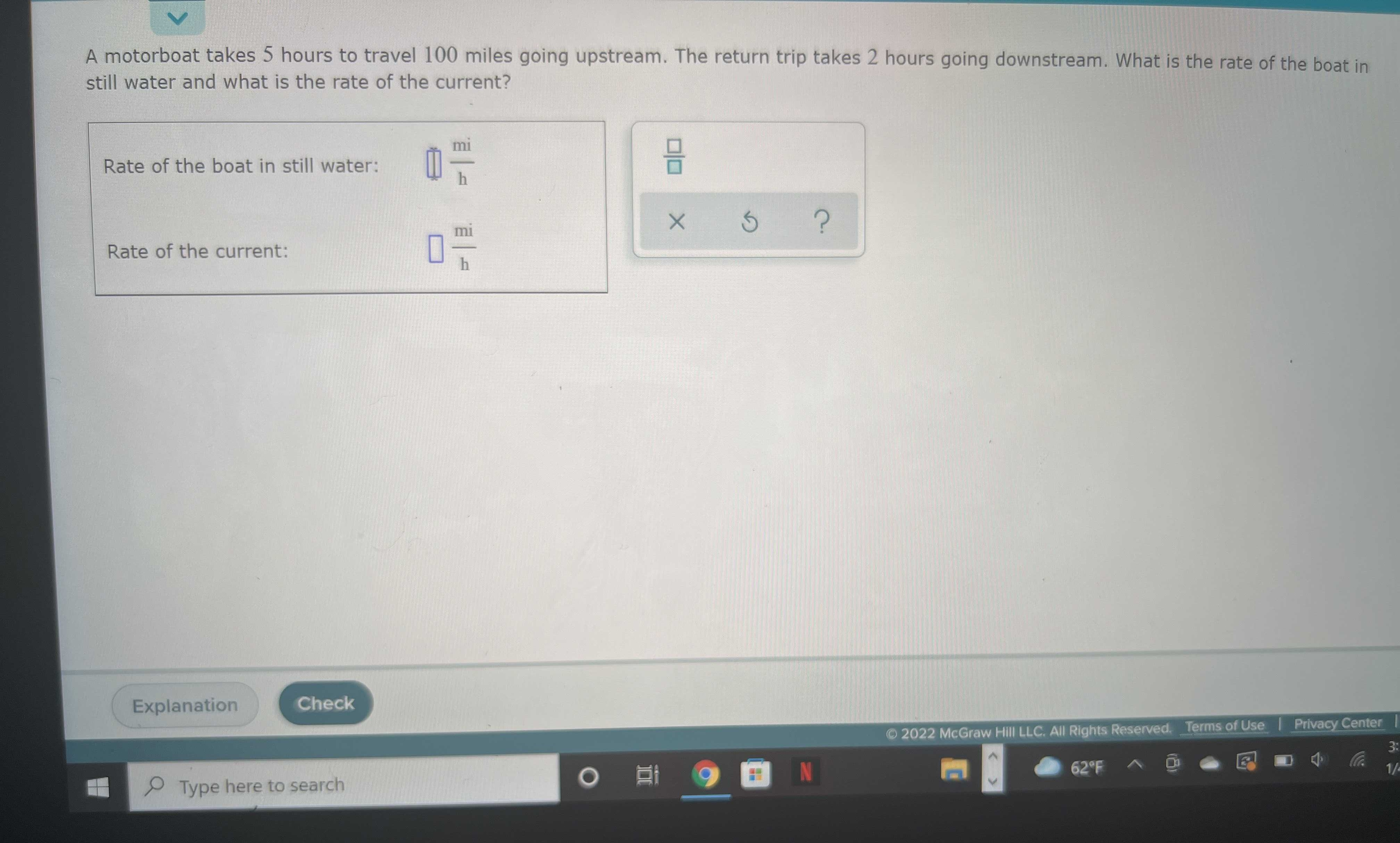### ¿Todavía tienes preguntas de matemáticas?

Pregunte a nuestros tutores expertos
Algebra
Pregunta

A motorboat takes $$5$$ hours to travel $$100$$ miles going upstream. The return trip takes $$2$$ hours going downstream. What is the rate of the boat in still water and what is the rate of the current?Rate of the boat in still water:  $$\square \frac { mi } { h }$$

Rate of the current:    $$\frac { mi } { h }$$